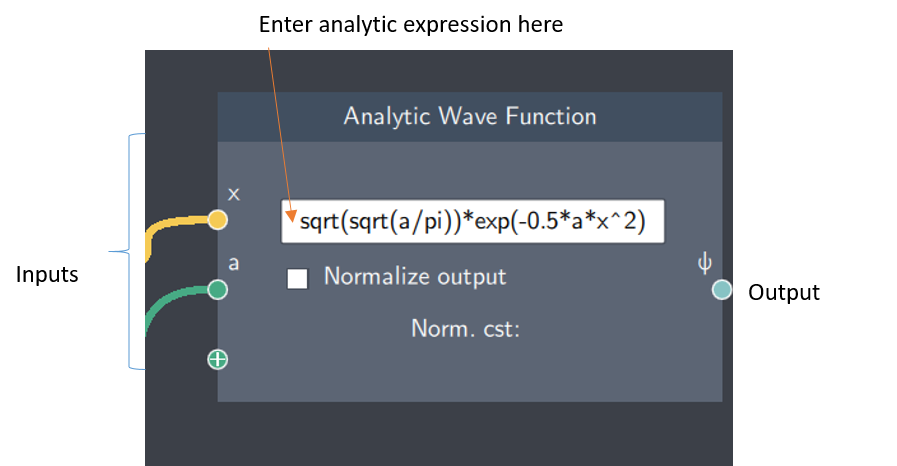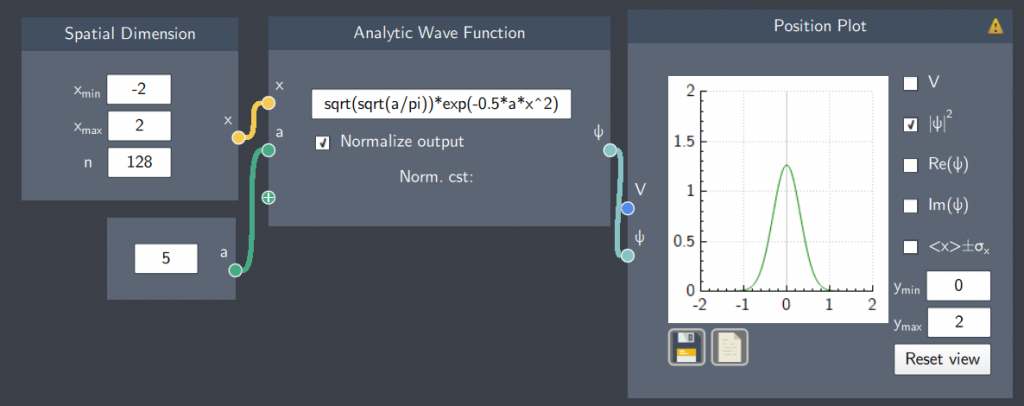# Analytic Wave Function

## Description

In this node, one can enter an analytic expression in the content field to define the wave function $\psi$.

An annotated screenshot of the node is shown below.## Inputs

The node has the following inputs:

• Spatial Dimension ($x$):  It inputs the values of $x$-axis defined in the Spatial Dimension node.
• Scalars ($a$, $b$ etc.) - It is possible to input scalar quantities to parameterize the expression.

## Content

In the node, any analytic expression can be written in the content field.  The wavefunction can be used either directly, or it can be numerically normalized by checking the ''Normalize output" checkbox which normalizes the output.

## Output

After all the inputs are provided, the node gives the following output:

• Analytic Wave Function ($\psi$) : It is the result of the defined expression based on its inputs.

## Example

An example is shown below where a gaussian function is defined with its inputs and the normalized output is shown in the plot.